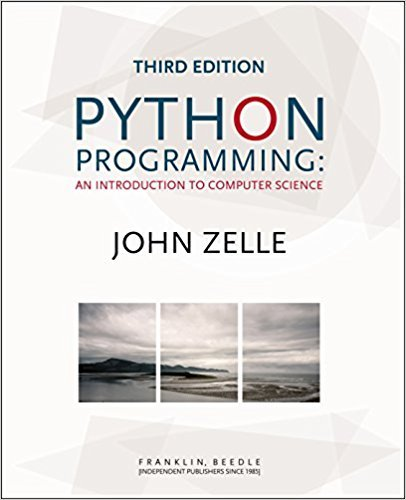×
×

# Redo the regression problem from Chapter 8 (Programming Exercise 13) using a RegressionISBN: 9781590282755 474

## Solution for problem 17 Chapter 10

Python Programming: An Introduction to Computer Science | 3rd Edition

• Textbook Solutions
• 2901 Step-by-step solutions solved by professors and subject experts
• Get 24/7 help from StudySoup virtual teaching assistantsPython Programming: An Introduction to Computer Science | 3rd Edition

4 5 1 252 Reviews
22
1
Problem 17

Redo the regression problem from Chapter 8 (Programming Exercise 13) using a Regression class. Your new class will keep track of the various quantities that are needed to compute a line of regression (the running sums of x, y, x2, and xy). The Regression class should have the following methods: _ _init__ Creates a new regression object to which points can be added. addPoint Adds a point to the regression object. predict Accepts a value of x as a parameter, and returns the value of the corresponding y on the line of best fit.

Step-by-Step Solution:
Step 1 of 3

Monday, November 9, 2015 BIOS 209 Week 10 Notes Ecology, Biomes, Global Climate (Interactions Between Organisms and Their Environment Include) -Abiotic environment: temperature precipitation social characteristics e.g., nutrients, texture water chemistry e.g., pH, salinity, DO -Biotic environment: competitors mates social...

Step 2 of 3

Step 3 of 3

##### ISBN: 9781590282755

Unlock Textbook Solution